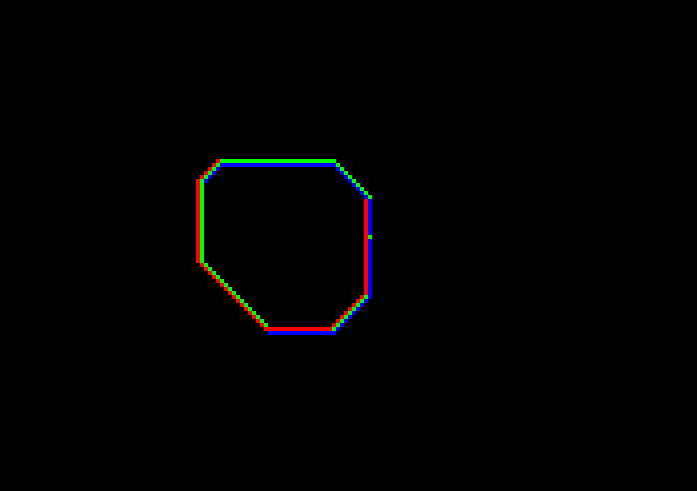# Using Input

Posted on December 10, 2015

I first ported a few more complicated examples from TONC, but I would rather introduce one thing at a time for these blog posts, so I’ve written a cute little etch-a-sketch example that’s based off of the first crate. We’re going to take input from the user and push the dots around.

It’s a little out of order compared to TONC, but you can check out the section on input for more details.

Here’s what I’ve done to main.rs. I’ve just moved the three points together, and created an x and a y to choose where to draw them.

pub extern "C" fn main(_: i32, _: *const *const i8) -> i32 {
let mut m = gfx::Mode3::new();

// Save our copy of the state of the keys.
let mut keys = input::Input::new();

// Location of the cursor.
let mut x : i32 = 120;
let mut y : i32 = 80;

// Avoid repeated typecasts.
let width = gfx::Mode3::WIDTH as i32;
let height = gfx::Mode3::HEIGHT as i32;

let colors = [Color::rgb15(31, 0, 0),
Color::rgb15(0, 31, 0),
Color::rgb15(0, 0, 31)];
loop {
// Wait for vsync, so we only draw once per frame.
gfx::vid_vsync();

// Save the current state of the keys.
// This keeps the previous state around,
// so we can check for button *presses*,
// and tell that apart from holding the button.
keys.poll();

// These are neat little helpers functions that encapsulate
// that pressing Left increases x and Right decreases it.
// tri_horz() will return -1, 0, or 1.

// This keeps everything positive.
// Note that just like as in C, -1 % 5 = -1, so we need to add width.
x = (x + width) % width;
y = (y + height) % height;

m.dot(x, y, colors);
m.dot((x+1) % width, y, colors);
m.dot((x+1) % width, (y+1) % height, colors);
}
}

We can then draw happy little loops:I also added the ability to cycle the colors around using the shoulder buttons.

    let mut color_ix = 0;
loop {
// ...
if keys.hit(Keys::L) {
color_ix -= 1;
} else if keys.hit(Keys::R) {
color_ix += 1;
}
color_ix = (colors.len() + color_ix) % colors.len();

// ...
m.dot(x, y, colors[color_ix]);
m.dot((x+1) % width, y,
colors[(color_ix + 1) % colors.len()]);
m.dot((x+1) % width, (y+1) % height,
colors[(color_ix + 2) % colors.len()]);
}

This uses keys.hit instead of keys.pressed or the tribool, so each time you press the button it shifts, but you have to release it before we can do it again.

Now, lets take a look at the input module that’s backing all this.


use ::memmap;

/// Keys also functions as the flags for the keys.
#[repr(C)]
#[derive(Copy, Clone, Debug)]
pub enum Keys {
A =      0x0001,
B =      0x0002,
Select = 0x0004,
Start  = 0x0008,
Right  = 0x0010,
Left   = 0x0020,
Up     = 0x0040,
Down   = 0x0080,
R      = 0x0100,
L      = 0x0200,
}

/// The OR of all the keys.
pub const KEY_MASK: u32 = 0x03FF;

//...

#[derive(Debug)]
pub struct Input {
prev: u32,
curr: u32,
}


We’ve got a nice flags enum in here, and an Input struct. This replaces the global in TONC, and we’ll just alocate it in main and pass it down to where it’s needed. Note that it’s not Copy, since we really don’t need it to be, and having a stale copy of it doesn’t actually seem too useful.

fn bit_tribool(bits: u32, negative : KeyIndex, positive : KeyIndex) -> i32{
((bits >> positive as u32) & 1) as i32
- ((bits >> negative as u32) & 1) as i32
}

impl Input {
/// You should only need one copy of this struct.
pub fn new() -> Input {
Input{ prev: 0, curr: 0}
}

/// This should be called once a frame.
pub fn poll(&mut self) {
self.prev = self.curr;
self.curr = unsafe {!(volatile_load(memmap::REG_KEYINPUT) as u32)}
}

/// hit checks if the key is now pressed, but wasn't before.
pub fn hit(&mut self, k: Keys) -> bool {
(!self.prev & self.curr) & (k as u32) != 0
}

// ...

/// These family of functions return -1, 0, or 1.
/// tri_horz is 1 when Left is pressed, and -1 when Right is.
pub fn tri_horz(&mut self) -> i32 {
bit_tribool(self.curr, KeyIndex::Left, KeyIndex::Right)
}
/// tri_vert is 1 when Up is pressed, and -1 when Down is.
pub fn tri_vert(&mut self) -> i32 {
bit_tribool(self.curr, KeyIndex::Up, KeyIndex::Down)
}

// ...
}

You can check out the full version of input.rs on github.

Lets take a closer look at .poll().

pub fn poll(&mut self) {
self.prev = self.curr;
self.curr = unsafe {!(volatile_load(memmap::REG_KEYINPUT) as u32)}
}
We save the current set of keys in prev, and then do a volatile_load from the REG_KEYINPUT register. We then immediately flip all the bits, since the GBA hardware actually clears the bits when buttons are pressed, which is weird, and we don’t want to have to think about that. We also mask it with the KEY_MASK, which is just the OR of all the key’s flags.
// memmap.rs
pub const REG_KEYINPUT: *mut u16 = (REG_BASE + 0x0130) as *mut u16;	// Key status